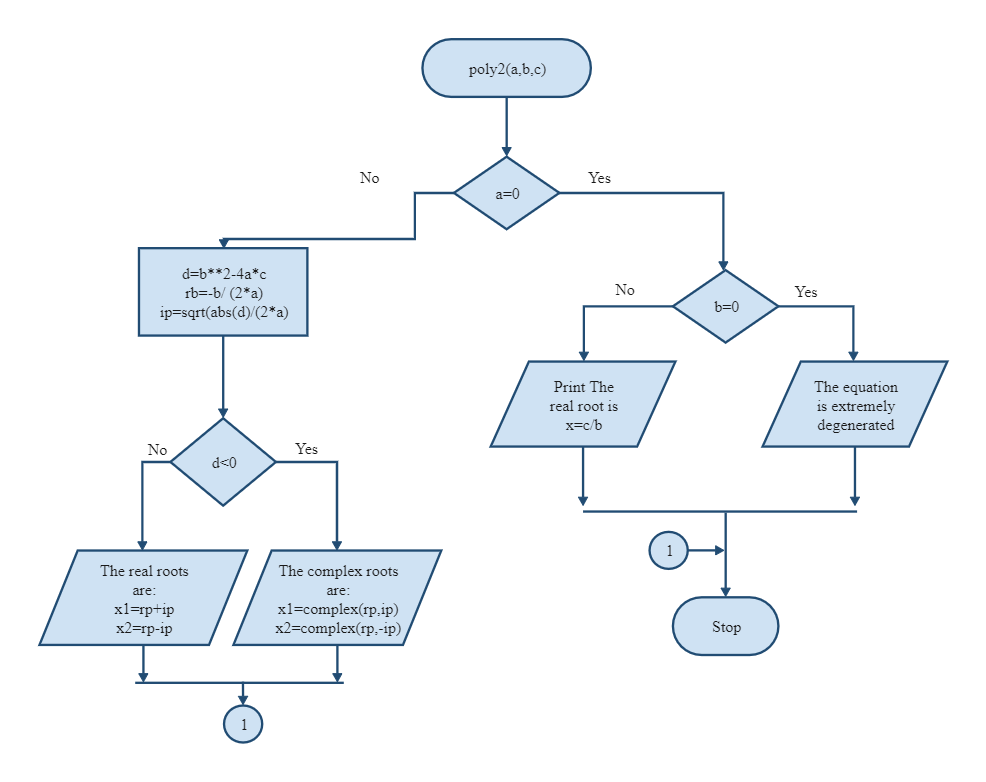Template Community / Root Algorithm Flowchart

# Root Algorithm FlowchartCharlotte
Published on 2021-07-16
Edit OnlineCreate an algorithm to understand the real roots and complex roots. Here in the diagram, if a=0 and b=0, no division or multiplication can happen, so the System will recognize it accordingly and stop the entire process. However, if a is not equals to zero, then the complex root will be identified by the mathematical equation: d=b**2-4a*c. However, if the value of d is less than zero, then the real roots will be identified by x1=rp+ip and x2=rp-ip. As the below illustration suggests, an algorithm is a series of instructions telling a system how to transform a set of facts about the world into useful information. The facts in this parameter where the calculation is happening are known as data, and the helpful information is knowledge for people, instructions for machines, or input for yet another algorithm.
Tag
algorithm flowchart
Flowchart Collection
Report
1
575PostRecommended Templates